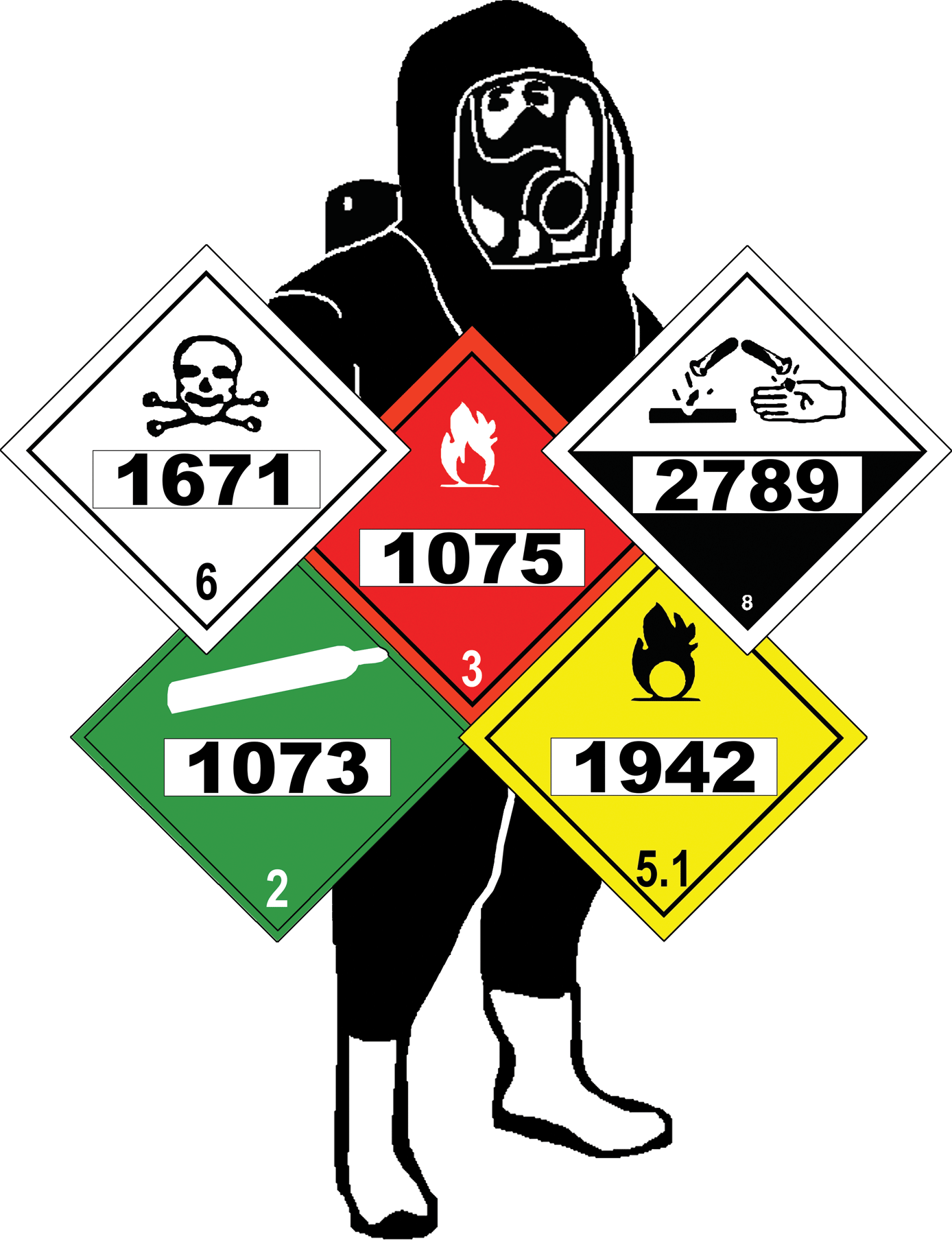# HAZMAT Operations Prep Exam

50 Questions | Total Attempts: 2147SettingsQuestions to help individuals practice for the dreaded Hazmat Operations Exam. This test may be taken as many times as you'd like, each time it will populate a random 50 questions from a 150+ question pool!

• 1.
What does APIE stand for?
• A.

Analyze, Plan, Implement, Evaluate

• B.

Analyze, Prepare, Investigate, Explore

• C.

• D.

• 2.
Select all that apply for Ops level task of Analyze
• A.

Predict likely behavior of a hazardous materials/WMD incident

• B.

Collect hazard and response information from SDS

• C.

Estimate the potentional harm at a hazardous materials/WMD incident

• D.

Survey incident to identify the containers and materials involved

• 3.
Describing emergency decon procedures is part of which Operation Level Responder task?
• A.

Analyze

• B.

Plan

• C.

Implement

• D.

Evaluate

• 4.
Operations Level Responder task, Implement, covers what responsibilty?
• A.

Establish and enforce scene control procedures including control zones, emergency decon, and communications

• B.

Develop a plan of action including safety considerations

• C.

Go downrange and inspect the area

• D.

Communicate the status of planned response

• 5.
What is the capacity of a Non Pressure Tank Car?
• A.

4,000 to 45,000 gallons

• B.

1,000 to 60,000 liters

• C.

20,000 to 100,000 gallons

• D.

55 gallons

• 6.
What is the pressure range of a Non Pressure Tank Car?
• A.

60 to 100 psi

• B.

12.5 to 25 psi

• C.

100 to 600 psi

• D.

40 to 80 psi

• 7.
Indentify:
• A.

Non pressure tank car

• B.

Pressure tank car

• C.

Intermodal Tank Car

• D.

Low pressure liquid Cargo Tank

• 8.
What is the pressure range of a Pressure tank car?
• A.

100 to 600 psi

• B.

60 to 80 psi

• C.

1000 psi

• D.

50 to 500 psi

• 9.
Indentify
• A.

Pressure tank car

• B.

Low pressure tank car

• C.

Cryogenic liquid tank car

• D.

Pressure intermodal

• 10.
Argon, Ethylene, Hydrogen, Nitrogen and Oxygen are commonly transport in which rail car?
• A.

Cryogenic Liquid tank car

• B.

Cryogenic intermodal tanks

• C.

Cryogenic bulk car

• D.

Cryogenic pressure tank

• 11.
Indentify:
• A.

Cryogenic liquid tank car

• B.

High pressure cargo car

• C.

Pressure tank car

• D.

Cryogenic intermodal

• 12.
IM 101 intermodal tank cars have which pressure range?
• A.

25.4 to 100 psi

• B.

14.5 to 25.4 psi

• C.

100 to 600 psi

• D.

1000 psi

• 13.
IM 102 Non-pressure intermodal tank cars have which pressure range?
• A.

14.5 to 25.4 psi

• B.

25.4 to 100 psi

• C.

1000 psi

• D.

100 to 200 psi

• 14.
Indentify
• A.

Non-pressure Intermodal

• B.

Pressure intermodal

• C.

• D.

Horizontal intermodal

• 15.
What is the capacity of Pressure intermodal tanks?
• A.

Up to 5,500 gallons

• B.

Up to 4,000 gallons

• C.

10,000 gallons

• D.

6,000 gallons

• 16.
Pressure intermodal tanks are designed to handle internal pressures ranging from what?
• A.

100 to 500 psi

• B.

500 to 1000 psi

• C.

60-100 psi

• D.

24.5 to 50 psi

• 17.
Indentify
• A.

Pressure intermodal tank

• B.

Cryogenic intermodal

• C.

Intermodal tube

• D.

Non pressure intermodal

• 18.
IMO type 7 describes which type of container?
• A.

Cryogenic intermodal tank

• B.

Cryogenic cargo tank

• C.

Cryogenic liquid tank car

• D.

Cryogenic dewer hickey

• 19.
Cryogenic intermodal tanks have a capacity of?
• A.

4,500 to 5,000 gallons

• B.

5500 to 10,000 gallons

• C.

1,000 to 5000 gallons

• D.

8.5M gallons

• 20.
Cryogenic intermodel tanks transport which hazard class?
• A.

2.2

• B.

4.8

• C.

1.4

• D.

5.2

• 21.
Identify:
• A.

Cryogenic intermodel

• B.

Horizontal tank

• C.

Non pressure intermodal

• D.

Fuel dispensing tank

• 22.
Pressure range of an intermodal tube container?
• A.

3,000 to 5,000 psi

• B.

1,000 to 3,000 psi

• C.

10,000 psi or more

• D.

2,500 psi

• 23.
Which one do you like?
• A.

Intermodal tube container

• B.

Stacked tube rail car

• C.

High pressure tank car

• D.

Cryogenic intermodal

• 24.
Which carries bulk fertilizers, oxidizers, grains, plastic products ect...
• A.

Dry Bulk Cargo / Pneumatic Hopper trailer

• B.

Dry cargo tank

• C.

Open top gondola

• D.

Dry bulk pressure hopper

• 25.
Indentify
• A.

Dry bulk cargo hopper

• B.

Dry bulk cargo tank

• C.Back to top